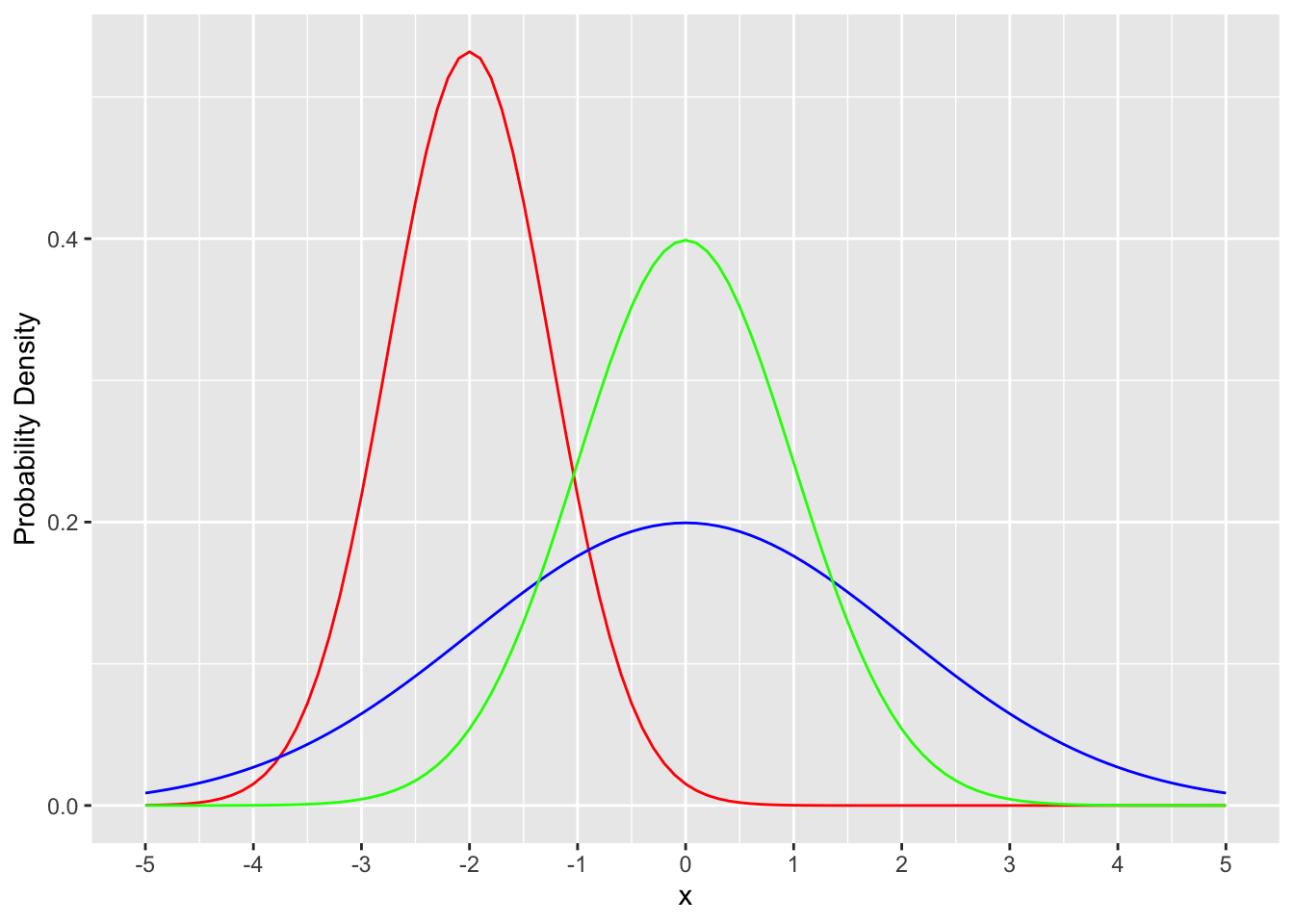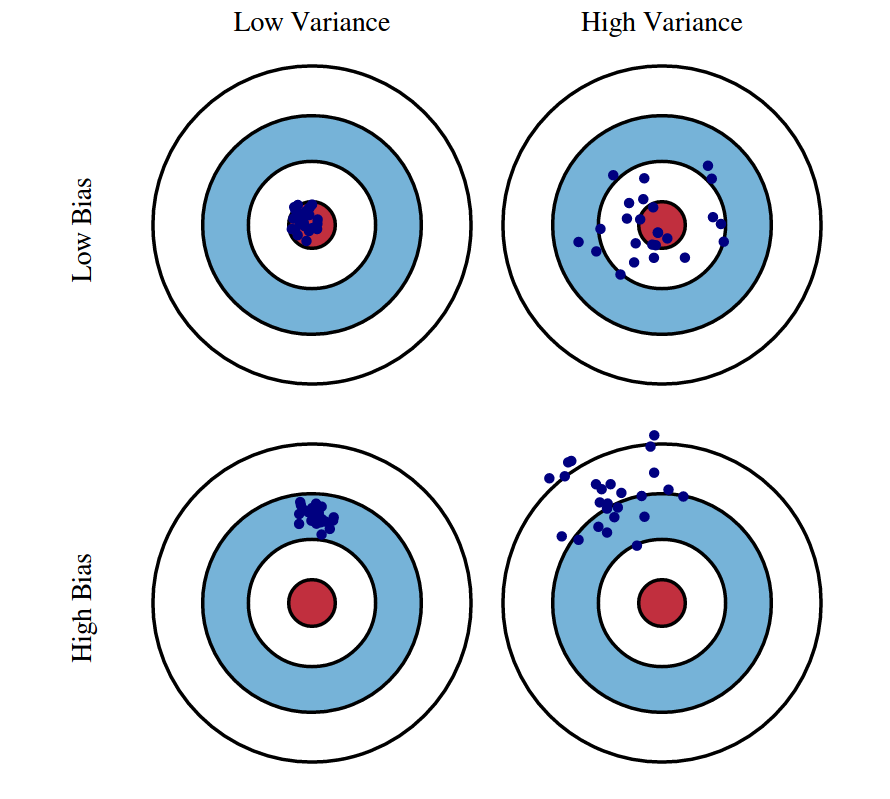WILD 502 schedule

Parameter Estimation from Samples

Samples

We typically observe systems incompletely, i.e., we sample. We then apply mathematical formulas to the data to obtain parameter estimates for quantities of interest.

Because only a sample is collected, estimates obtained are subject to sampling variability. For example, if we study annual survival rate for 10 animals from a population that has a true annual survival rate of 0.5, we might find that only 3 animals survive even though if we knew the true survival rate we would expected 5 of 10 to survive. Such a departure is due to sampling variability.

Distributions

We then think of natural variation in the organisms in terms of some underlying pattern that follow some frequency distribution (how common are the different values that can be observed). That frequency distribution reflects a probability distribution when individuals are sampled randomly.

Distributions can be broken into discrete distributions and continuous distributions. Within each of these types, we can further identify various distributions.

Some classic examples of discrete distributions include the binomial distribution (random events for which one of two outcomes can occur, e.g., live or die), or the multinomial distribution (random events for which >2 outcomes can occur, e.g., outcome of the the roll of a 6-sided die). Those two distributions will be used extensively in this course and you will become quite familiar with them.

Many data in biological samples are continuous in nature. For continuous data the probability distributions are smooth distribution functions over a range of values appropriate for the data. The most heavily used continuous distribution is the univariate normal distribution with probability density function.

$P(x | \mu, \sigma) = \frac{1}{{\sigma \sqrt {2\pi } }}\cdot e^{{{ - \left( {x - \mu } \right)^2 /{2\sigma ^2 }}}}$ The normal distribution is parameterized by its mean ($$\mu$$) and variance ($$\sigma^2$$). As you should know it is symmetric about its mean, bell-shaped, and more or less peaked depending on the variance. Below are 3 normal distributions for slightly different combinations (red: $$\mu = -2, \sigma = 0.75$$, blue: $$\mu = 0, \sigma = 2$$, green: $$\mu = 0, \sigma = 1$$).Distribution Parameters

The expected value of a random variable $$x$$ is the average of values that $$x$$ can take, with each value weighted by the frequency of occurrence of the value. For the roll of a die $$E(x) = 1(1/6) + 2(1/6) + … 6(1/6) = 3.5$$

Parameters of a distribution such as the mean or variance are typically not know but have to be estimated from sample data collected from the population. Thus, we do things like estimate the population mean based on the sample mean.

The theory of statistical inference deals with sample-based inferences about the parameters of a statistical population and the degree of confidence with which inferences can be made.

Replication and Statistical Independence

Replicate samples help us to better characterize a population and to assess the variability in our sampling procedure (how repeatable is it). Variance for values in a sample is calculated as follows.

$\sigma^2_x = \frac{\displaystyle\sum_{i=1}^{n}(x_i - \bar{x})^2} {n-1}$

Statistical independence occurs if the value of one random variable tells us nothing about the value of another beyond the fact that they were generated from the same random process.

When various random variables are not independent, they are correlated or covary, i.e., take values that are associated (either positively or negatively). Although most graduate students are familiar with correlation, many beginning students are less familiar with covariance.

$cov(x,y)=\frac{\sum_{i=1}^{n}(x_{i}-\bar{x})(y_{i}-\bar{y})}{n-1}$ Covariance values, unlike correlation values are not bounded between -1 and 1. Covariance relates to correlation as follows:

$corr(x_1,x_2) = \frac{cov(x_1,x_2)}{\sigma_{x_1}\cdot\sigma{_{x_2}}}$

Covariance is typically more informative if it is referenced to the underlying variation in each variable. Thus, one useful way of describing variables is via a variance-covariance matrix (sometimes called $$\boldsymbol{\Sigma}$$).

$\boldsymbol{\Sigma}=\begin{bmatrix}0.041 & 0.004 & -0.005 \\0.004 & 0.044 & -0.013 \\ -0.005 & -0.013 & 0.049 \end{bmatrix}$

The correlation matrix for this variance-covariance matrix is: $corr=\begin{bmatrix}1.000 & 0.094 & -0.112 \\0.094 & 1.000 & -0.280 \\ -0.112 & -0.280 & 1.000 \end{bmatrix}$

Probability Distributions - 2 Different Questions

1. The probability question – given a probability distribution with known parameters, how likely are various outcomes?

2. The estimation question – given observed data, what is the distribution from which the data arose? And, in our problems in this course, we’ll usually ask, “given observed data, what is (are) the corresponding value(s) of $$\theta$$ that parameterize the distribution that gave rise to those data.”

Bias, Precision, and Accuracy

If replicate samples were obtained, our estimates would vary and any given estimate will differ from other estimates and from the ‘true’ value of the parameter of interest. Thus, the question arises: how good is a given estimator in the sense of being close to the true parameter value. We measure this by considering estimator bias, precision, and accuracy.

Estimator Bias

If $$E(\theta)\ne\theta$$, then the estimator is biased with bias equal to the difference between $$E(\theta)$$ and $$\theta$$. Note, however, that bias is not measured by looking at one estimate resulting from the estimator but rather by the long-run behavior of the estimator.

It turns out that some estimators are, in fact, biased. Some estimators become quite biased if some of the assumptions underlying the estimator are violated, i.e., they are not robust to violations of some or all assumptions.

Precision

The tendency of replicated estimates to be dispersed is an expression of estimator precision, which is measured by the variance of the estimator: $$var(\hat\theta)=E([\hat\theta-E(\theta)]^2)$$.

Accuracy

Precision and bias do not have to be related, e.g., you can have a highly precise, biased estimate. Accuracy combines both bias and precision as an assessment of estimator performance. One method of doing so is mean squared error (MSE), which is defined as: $$MSE = var(\hat\theta) + bias(\hat\theta)^2$$.

Thus, an accurate estimator is precise and unbiased. An inaccurate estimator can be biased, imprecise, or both. Also, it is common in the statistics literature to refer to RMSE or the root mean squared error or the square root of MSE. Computer simulation or replicate samples are approaches used to evaluate estimator performance. You are probably familiar with images like this one that display the concepts with targets (original source: and image source).Estimation Procedures

We will use Maximum Likelihood Estimation throughout this course. It will be presented by using examples from the binomial distribution and later through the multinomial distribution. One presumes to know the mathematical form of the distribution function $$f(\underline{x}|\theta)$$, but not to know the actual value of $$\theta$$ for the distribution. We will express the likelihood function as $$L(\theta|\underline{x})$$, which emphasizes that we are trying to find the most likely parameter estimates given an observed dataset.

Confidence Intervals and Interval Estimation

How confident are you that the estimate obtained from random sampling accurately represents the actual parameter? We will address the question using confidence intervals for estimates. In a formal sense, we will seek to construct confidence intervals such that in replicated studies, confidence intervals about estimated parameters will include the true parameter value a stated percentage of the time. It is common to construct 95% confidence intervals: in such a case, if a study were repeated 100 times and a 95% CI were constructed each time, we would expect 95 of the 100 confidence intervals to contain the actual parameter value.

There are a variety of ways of constructing confidence intervals. One common method that we will encounter is the construction of asymptotically normal CIs: $$\hat\theta\underline{+}1.96\cdot\widehat{SE}(\hat\theta)$$.

The method is based on the MLE for the parameter being within 1.96 standard deviations of the parameter with probability 0.95. This approach relies on asymptotic properties of MLEs, but factors such as small sample sizes may cause CIs created this way to have coverage levels below the stated level. An alternative is to work with the actual likelihood profile to create CIs. CIs from the profile likelihood approach are often asymmetric about the estimated parameter value.

Model Evaluation

Goodness-of-Fit Tests

We would often like to be able to determine how adequate a statistical model is at characterizing our field data. For example, does a model that estimates a single annual survival rate adequate for describing survival obtained from male and female animals of varying ages from 5 different years? Here, we are evaluating a null hypothesis that the particular model being used fits the data. Rejection of the null hypothesis occurs if significant lack of fit is in evidence. We will use several goodness-of-fit (GOF) procedures in the course, learn of why one cares about GOF in model selection problems, and discuss what might be done in cases where lack of fit appears to be a problem.

Model Comparispons

We will be interested in comparing >2 candidate models as well as using GOF tests to assess the adequacy of models to characterize the data. In this course, we’ll use an information-theoretic approach that addresses the tradeoff between model fit and estimator variance using a statistic known as Akaike’s information criterion (AIC): $$AIC = -2lnL + 2k$$, where we’ll calculate an $$AIC$$ score for each model based on the log-likelihood ($$lnL$$) value for that model for the given data set and the number of parameters ($$k$$) in that model.

The idea is to select the model with the minimum AIC. We will see that there are modified versions of AIC that we can and will use. But for now the formula above will suffice. The key idea for now is that AIC emphasizes parsimony and makes a bias-variance tradeoff when evaluating competing models.

It is crucial when using AIC that all models being evaluated are fit to the same set of sample data. This may seem obvious but imagine a situation where you are comparing models S(yr,sex), S(yr), and S(sex) for 50 animals. Now imagine that during the trapping and radio-collaring operation that 5 animals were released before you could record the sex of the animal. In this case, the S(yr) model will be fit using 50 observations whereas the other 2 models will be fit to only 45 animals, i.e., the models are NOT all fit to the same set of sample data.

In some cases (and certainly not unusual cases!), no single model may be the clear selection, i.e., there are several models with AIC values that are similar and low. In such cases, model weights can be calculated based on the AIC scores. Such weights can be roughly interpreted as the probability that a given model is the best approximation to truth given the dataset and the model list under consideration. It is also possible to use the weights to obtain weighted averages of parameter estimates from across all models. The weights have appeal because they take into account the model-selection uncertainty inherent in the process.

Finally, note that:

1. in situations in which an experimental or quasi-experimental design provides a context for testing predictions based on theory, models, or both, it may be preferable to conduct a formal hypothesis test. Fortunately, in such contexts, MLE allows us to do such tests readily if they are desired.
2. there are plenty of alternatives to AIC, including Bayesian approaches, and a variety of information criteria, e.g., BIC, WAIC, DIC. We will occasionally discuss why one might consider some of the other methods.

We will be delving into the details of working with multi-model inference all semester, so know that much more information is coming your way!

WILD 502 schedule